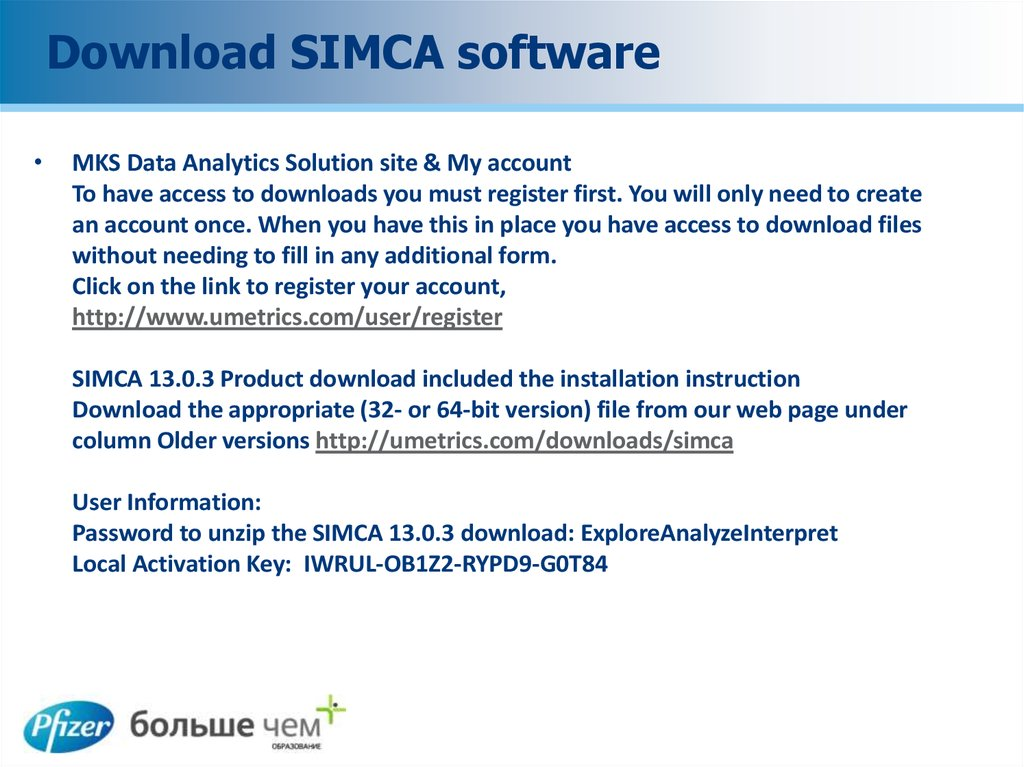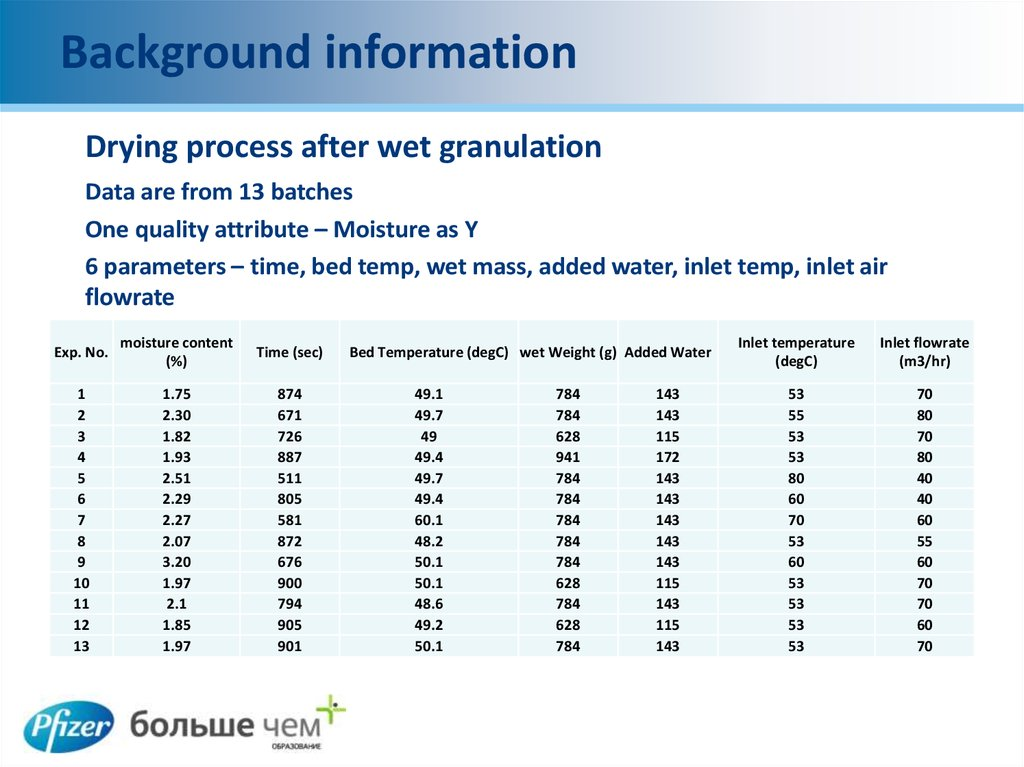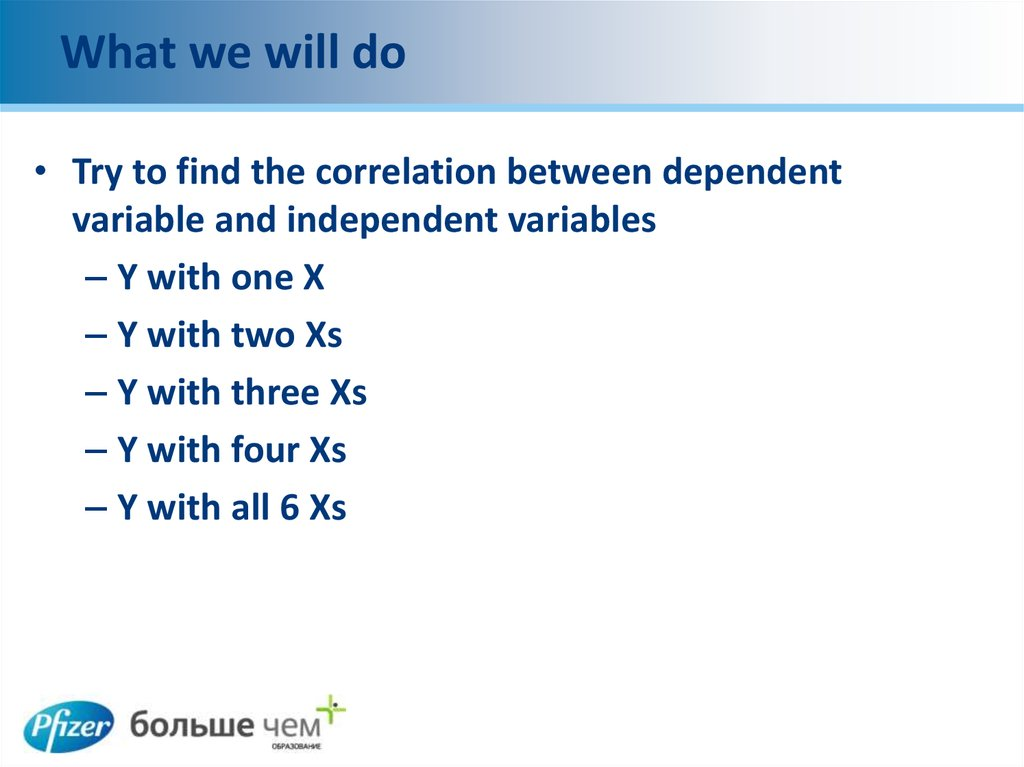MKS Data Analytics Solution site & My account
without needing to fill in any additional form.
http://www.umetrics.com/user/register
Download the appropriate (32- or 64-bit version) file from our web page under
User Information:
Local Activation Key: IWRUL-OB1Z2-RYPD9-G0T84

## 2. Background information

Drying process after wet granulation
Data are from 13 batches
One quality attribute – Moisture as Y
6 parameters – time, bed temp, wet mass, added water, inlet temp, inlet air
flowrate
Exp. No.
moisture content
(%)
Time (sec)
1
2
3
4
5
6
7
8
9
10
11
12
13
1.75
2.30
1.82
1.93
2.51
2.29
2.27
2.07
3.20
1.97
2.1
1.85
1.97
874
671
726
887
511
805
581
872
676
900
794
905
901
Bed Temperature (degC) wet Weight (g) Added Water
49.1
49.7
49
49.4
49.7
49.4
60.1
48.2
50.1
50.1
48.6
49.2
50.1
784
784
628
941
784
784
784
784
784
628
784
628
784
143
143
115
172
143
143
143
143
143
115
143
115
143
Inlet temperature
(degC)
Inlet flowrate
(m3/hr)
53
55
53
53
80
60
70
53
60
53
53
53
53
70
80
70
80
40
40
60
55
60
70
70
60
70

## 3. What we will do

• Try to find the correlation between dependent
variable and independent variables
– Y with one X
– Y with two Xs
– Y with three Xs
– Y with four Xs
– Y with all 6 Xs

## 4. Multivariable Regression

• Multivariable regression enables you to relate one
dependent variable to multiple independent variables
you've derived from measurements. This type of data
analysis helps you search for the effects of your
observed data on a related condition or predict a
condition based on other related observations.

## 5. Step 1

• Launch Microsoft Excel and click on the "File" tab in the
ribbon. Select "Options" to open the Excel Options dialog
box.

## 6. Step 2

• Click the "Add-Ins" item in the list on the left side of the
dialog box. You will see "Analysis ToolPak" in the list of
Inactive Application Add-Ins. Set the Manage drop-down
menu to "Excel Add-Ins," and click on the "Go" button at the
bottom of the dialog box to open the Add-Ins dialog box.

## 7. Step 3

• Tick the check box in front of "Analysis ToolPak" in the list of
available add-ins. Click the "OK" button on the right side of
the Add-Ins dialog box to turn on the Analysis ToolPak once
you have selected it in the list of options.

## 8. Step 4 & 5

Step 4 & 5
variable in the first or last column of your data. Use consecutive columns
for the data defining your independent variable's components.
• Switch to the "Data" tab in the Microsoft Excel ribbon and locate the
"Analysis" group. Click on the "Data Analysis" item to bring up the dialog
box of the same name

## 9. Step 6

• Scroll through the list of Analysis Tools until you locate
"Regression." Click on it to select it, and then click on the
"OK" button at the right to open its dialog box.

## 10. Step 7

Type the location of the cell range that contains your dependent variable into the
"Input Y Range" field in the Input section of the Regression dialog box. You can
click on the unlabeled "Collapse Dialog" button at the right edge of the field to
reduce the dialog box height temporarily so you can fill in the field by clicking on
the heading of the relevant data column. After you identify your data range, click
on the "Restore Dialog" button at the right edge of the input field to regain

## 11. Step 8

Enter the location of the cell range that contains your
independent variable – also called a predictor or explanatory
variable – into the "Input X Range" field.

## 12. Step 9 & 10

Step 9 & 10
• Click on the "Labels" check box to tell Excel that the first row of your
data contains data labels. If you didn't enter a header row, leave this box
unchecked.
• Click on the "Output Range" radio button in the Output Options section
of the dialog box and enter a data range in the entry field to identify a
location in your current worksheet for the output of your analysis. Use
the "Collapse Dialog" and "Restore Dialog" buttons to click through your
worksheet and identify where to put your results. Select the "New
Worksheet Ply" radio button to place your results in a new sheet within
your workbook, or choose "New Workbook" to put the results in a new
file.

## 13. Step 11

Choose options from the Residuals section of the Regression dialog box.
Residuals summarize the statistical output of the analysis on a case-by-case basis,
comparing the prediction derived from the regression equation to the difference
between it and the actual score. Standardized Residuals adjust the standard
deviation of Residuals to a value of 1. Tick the check box in front of the Plot
options to graph your results. The Residual Plot graphs your residuals, and the
Line Fit Plot compares the regression's prediction to its actual output.

## 14. Step 12

Click on the "OK" button at the right of the Regression dialog box to process your
regression. View your results in the location you specified, either on your current
worksheet, elsewhere in your document or in another file.
The first part of the output is the regression statistics
The ANOVA table comes next. This gives a test of significance of the R2.

## 15. Coefficients

The next stage is the coefficients. It gives the coefficient for each
parameter, including the intercept (the constant).
The standard errors, and the t-values follow (the t-value is the coefficient
divided by the standard error). Next comes the p-value associated with the
variable, and the confidence intervals of the parameter estimates

## 16. Different Plots

The Residual Plot graphs your residuals
The Line Fit Plot compares the regression's
prediction to its actual output.

## 17. Inserting a Scatter Diagram into Excel

Suppose you have two columns of data in Excel and you want to insert a
scatter plot to examine the relationship between the two variables.
• Begin by selecting the data in the two columns. Then, click on the Insert
tab on the Ribbon and locate the Charts section. Click on the button
labeled Scatter and then select the button from the menu titled Scatter
with Only Markers.

## 18. Add a Trendline to Excel

• You can now add your trendline. Begin by clicking once on any data point
in your scatter plot. This can be tricky because there are many elements
of the chart you can click on and edit. You will know that you have
selected the data point when all of the data points are selected. Once
you have selected the data points, right click on any one data point and

## 19. Formatting an Excel Trendline

To format your newly-created trendline, begin by right clicking on the line and
selecting Format Trendline from the menu. Excel will once again open up the
Format Trendline window
One of the more popular options people use when adding a trendline to Excel is
to display both the equation of the line and the R-squared value right on the
chart. You can find and select these options at the bottom of the window. For
now, select both of these options

## 20. Simple Linear Regression

Using an Excel function
Open the Microsoft Excel page with the data that you need to correlate. Name
one column "x" and the other "y". Select an empty cell below the columns that
will contain the correlation coefficient R, once calculated. Create a label above it
to distinguish it from other data cells.
Select the cell you created earlier and put an equal sign. Go to the Formula tab,
select Function Library Group, and then More Function and Statistical. Select
Slope, a dialog box will pop up asking you to infill the range.

## 21. Simple Linear Regression

In array one, fill in the number of variable (named x) Repeat
the procedure for array Y. Close the dialog box by clicking
OK. The result will be displayed in the cell

## 22. Linear Regression Formula

Repeat the same process to get result for Intercept - Correlation
and R- Squared
The syntax to calculate each of the terms in the regression is as
follows:
• The SLOPE function returns the slope of the linear regression
line that is used to predict Y values from X values.
Slope,m: =SLOPE(Known_Y’s,Known_x’s)

## 23. Linear Regression Formula

• The intercept point is based on a best-fit regression line
plotted through the known x-values and known y-values.
Calculates the point at which a line will intersect the y-axis
by using existing x-values and y-values.
Y-intercept,b: =INTERCEPT(Known_Y’s,Known_x’s)
• The correlation coefficient (a value between -1 and +1) tells
you how strongly two variables are related to each other. We
can use the CORREL function or the Analysis Toolpak add-in
in Excel to find the correlation coefficient between two
variables
Correlation Coefficient, r: =CORREL(Known_Y’s,Known_x’s)
• R-squared, r2 =RSQ(Known_Y’s,Known_x’s)